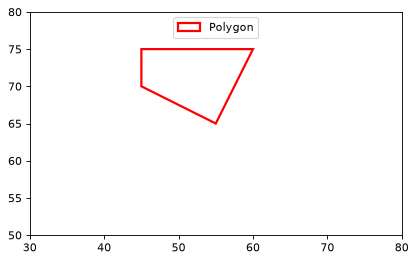# PolygonPixelRegion¶

class regions.PolygonPixelRegion(vertices, meta=None, visual=None)[source]

A polygon in pixel coordinates.

Parameters: vertices : PixCoord The vertices of the polygon meta : RegionMeta object, optional A dictionary which stores the meta attributes of this region. visual : RegionVisual object, optional A dictionary which stores the visual meta attributes of this region.

Examples

import numpy as np
from astropy.coordinates import Angle
from regions import PixCoord, PolygonPixelRegion
import matplotlib.pyplot as plt

x, y = [45, 45, 55, 60], [75, 70, 65, 75]
fig, ax = plt.subplots(1, 1)

vertices = PixCoord(x=x, y=y)
reg = PolygonPixelRegion(vertices=vertices)
patch = reg.as_artist(facecolor='none', edgecolor='red', lw=2)

plt.xlim(30, 80)
plt.ylim(50, 80)
ax.set_aspect('equal')Attributes Summary

 area Region area (float). bounding_box The minimal bounding box (in integer pixel coordinates) that contains the region. vertices Descriptor class for PixelRegion which takes a one dimensional regions.PixCoord object.

Methods Summary

 as_artist(self[, origin]) Matplotlib patch object for this region (matplotlib.patches.Polygon). contains(self, pixcoord) Checks whether a position or positions fall inside the region. rotate(self, center, angle) Make a rotated region. to_mask(self[, mode, subpixels]) Returns a mask for the aperture. to_sky(self, wcs) Returns a region defined in sky coordinates.

Attributes Documentation

area

Region area (float).

bounding_box

The minimal bounding box (in integer pixel coordinates) that contains the region.

vertices

Descriptor class for PixelRegion which takes a one dimensional regions.PixCoord object.

Methods Documentation

as_artist(self, origin=(0, 0), **kwargs)[source]

Matplotlib patch object for this region (matplotlib.patches.Polygon).

Parameters: origin : array_like, optional The (x, y) pixel position of the origin of the displayed image. Default is (0, 0). kwargs : dict All keywords that a Polygon object accepts patch : Polygon Matplotlib polygon patch
contains(self, pixcoord)[source]

Checks whether a position or positions fall inside the region.

Parameters: pixcoord : PixCoord The position or positions to check.
rotate(self, center, angle)[source]

Make a rotated region.

Rotates counter-clockwise for positive angle.

Parameters: center : PixCoord Rotation center point angle : Angle Rotation angle region : PolygonPixelRegion Rotated region (an independent copy)
to_mask(self, mode='center', subpixels=5)[source]

Returns a mask for the aperture.

Parameters: mode : { ‘center’ | ‘exact’ | ‘subpixels’}, optional The following modes are available: 'center': returns 1 for pixels where the center is in the region, and 0 otherwise. 'exact': returns a value between 0 and 1 giving the fractional level of overlap of the pixel with the region. 'subpixels': A pixel is divided into subpixels and the center of each subpixel is tested (a subpixel is either completely in or out of the region). Returns a value between 0 and 1 giving the fractional level of overlap of the subpixels with the region. With subpixels set to 1, this method is equivalent to 'center'. subpixels : int, optional For the 'subpixel' mode, resample pixels by this factor in each dimension. That is, each pixel is divided into subpixels ** 2 subpixels. mask : Mask A region mask object.
to_sky(self, wcs)[source]

Returns a region defined in sky coordinates.

Parameters: wcs : WCS instance The world coordinate system transformation to assume sky_region : SkyRegion object.